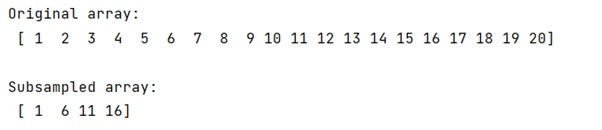# Subsampling every nth entry in a NumPy array

Learn how to subsample every nth entry in a NumPy array in Python?
Submitted by Pranit Sharma, on December 28, 2022

NumPy is an abbreviated form of Numerical Python. It is used for different types of scientific operations in python. Numpy is a vast library in python which is used for almost every kind of scientific or mathematical operation. It is itself an array which is a collection of various methods and functions for processing the arrays.

## NumPy Array: Subsampling Every Nth Entry

Suppose we need to extract some data from a long numpy array, we will start from a defined position in the array, and then subsample every nth data point from that position, until the end of the array.

For example, if we have an array of 20 elements and we start from arr and we need to subsample every 5th element of this array.

For this purpose, we will use the numpy slicing technique. There is a common approach of slicing and repeating the elements after some steps.

Start:Stop:Step fashion allows us to solve this problem.

Let us understand with the help of an example,

## Python code to subsample every nth entry in a NumPy array

```# Import numpy
import numpy as np

# Creating a numpy array
arr = np.array(
[1,2,3,4,5,6,7,8,9,10,
11,12,13,14,15,16,17,18,19,20]
)

# Display original array
print("Original array:\n",arr,"\n")

# Slicing every 5th element and starting
# from 1st element
res = arr[0::5]

# Display result
print("Subsampled array:\n",res,"\n")
```

Output: